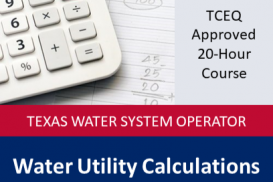Core Courses
Water Utility Calculations (1329)
The Water Utility Calculations course is intended to provide the necessary training for Texas Water and Wastewater operators to quickly and accurately make calculations necessary to properly operate water and wastewater treatment plants and system...
View more
Course content
• Course Description and Learning Objectives
• Course Description and Learning Objectives
• Course References
• TCEQ Approved Training Manual Link
• Course Completion Tutorial
• Basic Water Utility Calculations
• Unit Conversions
• Working with Formulas
• Understanding Percentages
• Calculating Area
• Calculating Volume
• Weight-Volume Relationships
• Velocity and Flow Rate
• Pumps
• Problem Solving
• Distribution System Calculations
• Flow Conversion Problems
• Volume Problems
• Flow Rate Problems
• Pipe Flow and Replacement Problems
• Distribution Disinfection Problems
• Ground Water Production and Treatment Calculations
• Groundwater Production Problems
• Chemical Feed Problems
• Surface Water Treatment Calculations
• Reservoir Problems
• Chemical Dose Problems
• Coagulation and Flocculation Problems
• Sedimentation Problems
• Filtration Problems
• Disinfection Problems
• Wastewater Treatment Calculations
• Grit Channel Problems
• Clarifier Problems
• Pond Problems
• Trickling Filter Problems
• Wastewater Disinfection Problems
• Anaerobic Sludge Digestion Problems
• Activated Sludge Problems